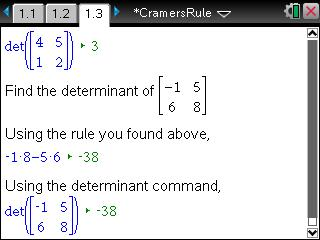# Activities

••• ##### Subject Area

• Math: Algebra II: Matrices
• Math: Algebra II: Systems of Linear Equations and Inequalities
• Math: College Algebra: Linear Systems and Matrices

• ##### Author9-12

30 Minutes

• ##### Device
•TI-Nspire™ CX/CX II
•TI-Nspire™ CX CAS/CX II CAS
• TI-Nspire™ Navigator™
• TI-Nspire™
• TI-Nspire™ CAS
• ##### Software

TI-Nspire™
TI-Nspire™ CAS

3.0

## Cramer's Rule

#### Activity Overview

Students are introduced to determinants of two-by-two matrices by discovering that the determinant can be found by subtracting the products of the diagonals. Students are then introduced to Cramer's Rule and use it to solve three systems that have one solution, no solutions, and an infinite number of solutions. They graph the equations to verify their answers and are asked how Cramer's Rule can be used to determine the number of solutions to a system of linear equations.

#### Key Steps

•A two-by-two matrix and its determinant are shown on page 1.3. Let students discover the rule to calculate a determinant by changing different entries in the matrix and observing the changing values of the determinant.